IMO Shortlist 2014 problem N7

Kvaliteta:
Avg: 0,0
Težina:
Avg: 9,0

Let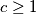$c \geq 1$ be an integer. Define a sequence of positive integers by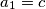$a_1 = c$ and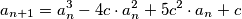for all$n \geq 1$. Prove that for each integer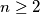$n \geq 2$ there exists a prime number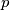$p$ dividing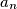$a_n$ but none of the numbers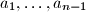$a_1, \ldots, a_{n-1}$.

(Austria)

Izvor: https://www.imo-official.org/problems/IMO2014SL.pdf